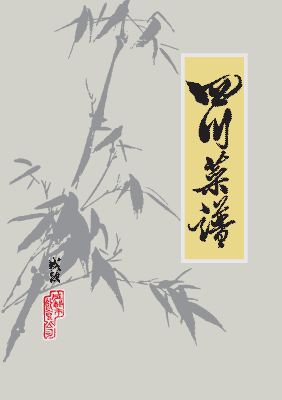\$14.99

\$24.99

# 关于本书

《四川菜谱》是成都市饮食公司所编纂，于一九七二年七月发行的内部资料。其中记述了三百一十二个菜品。随着时间流逝，市面餐馆日常出品的一些菜品已经不同于传统口味。很多菜品更是鲜少出现在现今的餐桌上。更有一些稀有的原料已经消失不见。为了承传历史，特将这本1972年编印的《四川菜谱》整理、修订、重制，以电子书的形式重新发布。

• ### 分类

• Food & Drink
• Culture

Email 给作者（们）

• 肉食类
• 一、回锅肉
• 二、鱼香肉片
• 三、小滑肉
• 四、干煵肉丝
• 五、陈皮肉
• 六、锅巴肉片
• 七、水煮肉片
• 八、旱蒸回锅肉
• 九、芙蓉肉片
• 一〇、萝卜连锅
• 一一、粉蒸肉
• 一二、咸烧白
• 一三、夹沙肉
• 一四、龙眼咸烧白
• 一五、龙眼甜烧白
• 一六、荷叶蒸肉
• 一七、大南瓜蒸肉
• 一八、芙蓉杂烩
• 一九、奶汤大杂烩
• 二〇、松子肉
• 二一、晾干肉
• 二二、麻圆肉
• 二三、鹅黄肉
• 二四、松花肉
• 二五、南卤肉
• 二六、双色肉糕
• 二七、芙蓉肉糕
• 二八、焦皮肘子
• 二九、烧皱皮肉
• 三〇、芝麻肘子
• 三一、罐烧肉
• 三二、红枣煨肘
• 三三、南煎圆子
• 三四、苕菜狮子头
• 三五、软炸蒸肉
• 三六、软炸子盖
• 三七、炸扳指
• 三八、软炸肚头
• 三九、软炸腰卷
• 四〇、锅贴腰片
• 四一、清汤腰方
• 四二、兰花肚丝
• 四三、葱末肝片
• 四四、白油猪肝
• 四五、糊辣腰块
• 四六、肥肠豆沙汤
• 四七、竹荪肝膏汤
• 四八、菠饺白肺
• 四九、菠饺玻璃肚
• 五〇、四上玻璃肚
• 五一、龙眼脊髓
• 五二、红烧环喉
• 五三、生烧筋尾舌
• 五四、豆渣猪头
• 五五、罈子肉
• 五六、烤酥方
• 五七、叉烧奶猪
• 鸡鸭类
• 五八、怪味鸡
• 五九、棒棒鸡丝
• 六〇、豆芽拌鸡丝
• 六一、自拌鸡丝
• 六二、热窝姜汁鸡
• 六三、辣子鸡丁
• 六四、糊辣鸡丁
• 六五、花椒鸡丁
• 六六、陈皮鸡
• 六七、熘珊瑚鸡丁
• 六八、熘鸡米
• 六九、家常鸡丝
• 七〇、兰花鸡丝
• 七一、贵州鸡
• 七二、海椒香辣鸡
• 七三、旱蒸全鸡
• 七四、三菌炖鸡
• 七五、生烧鸡翅
• 七六、生烧鸡腿
• 七七、油淋仔鸡
• 七八、福建仔鸡
• 七九、香炸仔鸡
• 八〇、五香脆皮鸡
• 八一、雪花鸡淖
• 八二、鱼香八块鸡
• 八三、鸡淖脊髓
• 八四、芙蓉鸡片
• 八五、三色鸡淖
• 八六、鸡豆花
• 八七、牡丹鸡片
• 八八、羊耳鸡卷
• 八九、网油鸡卷
• 九〇、鸡塔
• 九一、锅贴鸡片
• 九二、熘桃鸡卷
• 九三、麻酥鸡
• 九四、五彩鸡片
• 九五、刷把鸡丝
• 九六、红烧卷筒鸡
• 九七、软炸鸡糕
• 九八、桃酥鸡糕
• 九九、糯米鸡
• 一〇〇、泥糊鸡
• 一〇一、包烧鸡
• 一〇二、魔芋烧鸭
• 一〇三、姜爆鸭丝
• 一〇四、锅烧全鸭
• 一〇五、香酥鸭子
• 一〇六、银杏鸭脯
• 一〇七、红烧鸭卷
• 一〇八、虫草鸭子
• 一〇九、冷汁盐水鸭
• 一一〇、抄手鸭子
• 一一一、南边鸭子
• 一一二、酿鸭脯
• 一一三、豆渣鸭脯
• 一一四、豆渣鸭子
• 一一五、樟茶鸭子
• 一一六、糯米全鸭
• 一一七、蛋酥鸭子
• 一一八、熘鸭肝
• 一一九、醉鸭肝
• 一二〇、竹荪双脆
• 一二一、汆双脆
• 一二二、红烧舌掌
• 一二三、麻辣?松
• 一二四、鸭腰莼菜
• 一二五、软炸鸭腰
• 鱼虾类
• 一二六、芹黄鱼丝
• 一二七、兰花熘鱼片
• 一二八、清蒸鲢鱼
• 一二九、辣子鱼
• 一三〇、泡菜鱼
• 一三一、豆腐鲫鱼
• 一三二、糖醋脆皮鱼
• 一三三、干烧鲫鱼
• 一三四、凉粉鲫鱼
• 一三五、烟熏鱼
• 一三六、葱酥鱼
• 一三七、白汁五柳鱼
• 一三八、白汁鲜鱼
• 一三九、萝卜丝中段
• 一四〇、干煸鳝鱼
• 一四一、清蒸青鳝
• 一四二、红烧甲鱼
• 一四三、黄焖大鲢鱼头
• 一四四、五柳鱼
• 一四五、松鼠鱼
• 一四六、清汤鱼卷
• 一四七、五色鱼圆
• 一四八、椒盐鱼卷
• 一四九、锅贴鱼片
• 一五〇、炸熘鱼圆
• 一五一、清汤五彩鱼
• 一五二、包烧鱼
• 一五三、南卤醉虾
• 一五四、生爆虾仁
• 一五五、清烩虾仁
• 一五六、翡翠虾仁
• 一五七、芙蓉虾仁
• 一五八、番茄炒虾仁
• 一五九、椒盐虾饼
• 一六〇、软炸虾包
• 一六一、软炸虾糕
• 一六二、芝麻虾
• 一六三、五色虾球
• 山海味类
• 一六四、凉拌麂肉
• 一六五、红烧雪猪
• 一六六、红烧鹿筋
• 一六七、红烧熊掌
• 一六八、清炖鹿冲
• 一六九、佛手蜇卷
• 一七〇、干煸鱿鱼笋丝
• 一七一、鸡皮鱼肚
• 一七二、干煸鱿鱼丝
• 一七三、家常海参
• 一七四、鱼羊肚烩
• 一七五、鱿鱼腐皮
• 一七六、鲜花海参丝
• 一七七、肝油海参
• 一七八、鸡淖鱼翅
• 一七九、红烧鱼唇
• 一八〇、清汤鱼翅把
• 一八一、干烧鱼翅
• 一八二、玻璃鱿鱼
• 一八三、菠饺鱼肚
• 一八四、清汤鱼肚卷
• 一八五、棋盘鱼肚
• 一八六、桂花珧柱
• 一八七、酿海参
• 一八八、绣球珧柱
• 一八九、菊花珧柱
• 一九〇、白酿海参
• 一九一、绣球鱼翅
• 一九二、凤尾鱼翅
• 一九三、荷包鱿鱼
• 一九四、佛手海参
• 一九五、蝴蝶海参
• 甜食类
• 一九六、冰糖银耳
• 一九七、糖粘羊尾
• 一九八、酥扁豆泥
• 一九九、玫瑰锅炸
• 二〇〇、蜜味锅蒸
• 二〇一、酥蚕豆泥
• 二〇二、蒸枣糕
• 二〇三、网油枣卷
• 二〇四、蜜汁苕蛋
• 二〇五、糯米圆子
• 二〇六、茨菰饼
• 二〇七、酿藕
• 二〇八、水晶球
• 蔬菜类
• 二〇九、烧拌鲜笋
• 二一〇、鸡皮慈笋
• 二一一、干煸冬笋
• 二一二、盐白菜冬笋
• 二一三、鸡皮冬笋
• 二一四、四吃露笋
• 二一五、蟹黄凤尾
• 二一六、炸豆芽饼
• 二一七、酿萝卜
• 二一八、鱼香茄饼
• 二一九、白汁菠菜卷
• 二二〇、清汤三色卷
• 二二一、五色卷
• 二二二、碎米豆腐
• 二二三、蜂窝豆腐
• 二二四、双色豆腐淖
• 二二五、韭汁豆蕊
• 二二六、椒盐豆腐糕
• 二二七、白汁豆腐饼
• 二二八、格花豆腐皮
• 二二九、菱角豆腐
• 二三〇、锅贴豆腐
• 二三一、口袋豆腐
• 二三二、鸡蒙葵菜
• 二三三、清汤白菜
• 其它类
• 二三四、椿芽烘蛋
• 二三五、陈皮兔
• 二三六、熏牛肉
• 二三七、兰花田鸡
• 二三八、鸡蒙兰花
• 二三九、家常田鸡
• 二四〇、白汁蛋饺
• 二四一、炸玉兰
• 二四二、炸荷花卷
• 二四三、蟹黄银杏
• 二四四、火把玉兰片
• 二四五、酸辣虾羹汤
• 二四六、红烧鸽子
• 二四七、凤尾鸽蛋
• 二四八、凤眼鸽蛋
• 二四九、酿鸽蛋
• 二五〇、筋尾鸽蛋
• 二五一、芙蓉月光鸽蛋
• 二五二、蹄燕鸽蛋
• 二五三、子母烩
• 二五四、叉烧宣腿
• 二五五、炸熘田鸡腿
• 二五六、五彩吐司
• 二五七、兰花吐司
• 二五八、杏圆吐司
• 二五九、酿冬菇
• 二六〇、软炸口蘑
• 二六一、海味什锦
• 二六二、生片菊花锅
• 二六三、蝴蝶竹荪
• 二六四、酿两样
• 重庆市部分菜肴
• 烧牛头方
• 烟熏排骨
• 炸扳指
• 冬菜蒸肉饼
• 清汤竹荪肝膏
• 冬菜腰片汤
• 锅贴肚头
• 干烧岩鲤
• 旱蒸鱼
• 锅贴鱼
• 叉烧全鸡
• 烟熏仔鸡
• 椒麻鸡
• 姜片鸭子
• 锅贴鸭方
• 熘黄菜
• 火腿烘蛋
• 干煸冬笋
• 酿茄饼
• 核桃泥
• 酿梨
• 传丝杂烩汤
• 清蒸肥头鱼
• 炒鸭脯
• 虫草蒸鸭
• 红烧鸭卷
• 叉烧火腿
• 奶汤素烩
• 网油黄秧白
• 干贝烧冬苋菜
• 豆渣烘猪头
• 罈子肉
• 椒盐蹄膀
• 干煸牛肉丝
• 半汤鱼
• 菜鱼汤
• 醋熘鸡
• 格呢仔鸡
• 家常鸡
• 芙蓉鸡片
• 芹黄烧拌冬笋
• 炒杂瓣
• 小煎鸡
• 口袋豆腐
• 清炖牛肉汤
• 清炖牛尾汤
• 清炖枸杞牛鞭汤
• 炒牛肚梁